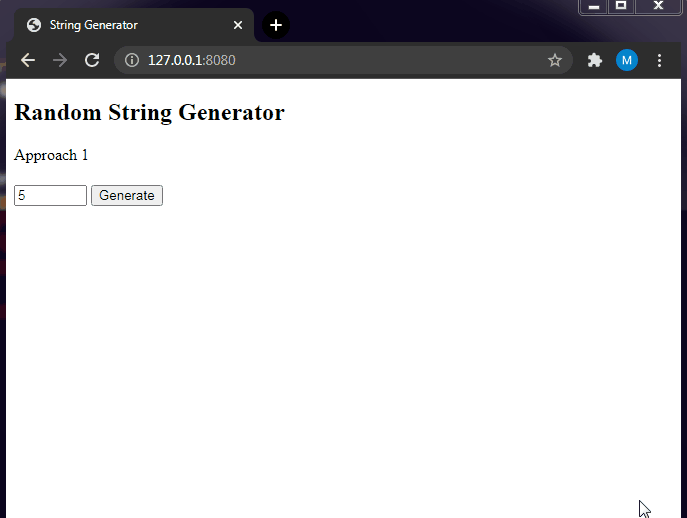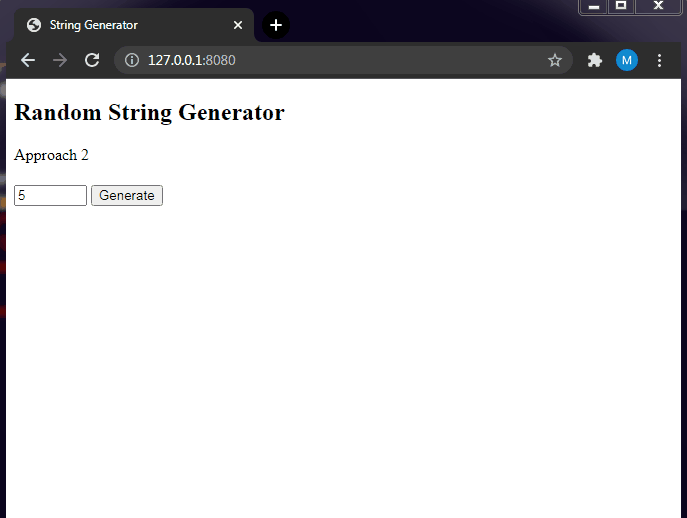# Random String Generator using JavaScript

• Difficulty Level : Medium
• Last Updated : 23 Nov, 2021

JavaScript is a lightweight, cross-platform, interpreted scripting language. It is well-known for the development of web pages, many non-browser environments also use it. JavaScript can be used for Client-side developments as well as Server-side developments.

In this article, we need to create a Random String Generator using Javascript that will generate a random set of strings & get displayed when the user clicks a button. Additionally, we can also take input for the length of the string from the user. On the client-side, it will render those strings once generated using the Document Object Model (DOM) concept.

Approach: For generating Random Strings in Javascript, we can use in-built methods that will actually generate the strings and can manipulate the Document Object Model (DOM) accordingly. We will use the Math library to access the random() function that will help to generate the random index and multiple with the length of the string ie., it will append the character from the string or character set as it is passed.

We can generate the random string in 2 ways ie., either we can iterate the loop for the specified length of the character set or we can use String.fromCharCode() method that will convert the UTF-16 codes into their equivalent characters and returns the string. By using the 2nd approach, we can manually take input for the length of the string from the user then generate the string of that particular length by accessing the DOM elements. For both approaches, we will use the Math.random() function.

Generating Random Strings: Here, we will generate strings only with lowercase letters characters. Let’s start by discussing the two different approaches for generating the strings.

Approach 1: Generating the random string from the specified length of the character set:

• Declare the character set that will use for generating string.
• Access the length of that character set from the input.
• Construct the iteration loop for random character generation.
• Use Math functions in javascript & assign them to the variable.

We will utilize the above concepts & generate the strings randomly of user-defined length using Javascript and DOM manipulation.

Defining the character set: We have defined a variable “characters” that will contain all the characters as we require. We will use another variable “result” which is initialized as an empty string.
Accessing the length of the string: After this, we will fetch the length of the string that we need to generate by using the document.getElementById method to access the HTML element or the user input field that contains the length.

`length = document.getElementById("strlength").value;`

So, we have used the “strlength” id which is declared inside the  HTML <input> tag. We can get the value of the element using the “.value” attribute and then assign it to a “length” variable. Thus, we have now obtained the length of the string that is needed to generate.

Generating Random characters: We will use a for loop to generate n(length) number of characters and append those to the string. The loop will run from 0 till length -1 as the index value start from 0.

```for ( let i = 0; i < length; i++ ) {
// Code
}```

Now, we will use the Math.random() function to generate a random index in the string. Before that, we need to get the length of the “characters” string and store it in a const variable “charactersLength”. This will give us the length of the “charactersLength” string which is 26. Now, using the charAt() function that will return the character at a specified index in a string & we can get the random number.

The Math.random() function will give the value between 0 & 1 ie., decimal value & we need to round it off to get an integer value. Hence, we use Math.floor to round off the decimal value. We will multiply the Math.random() function with the variable charactersLength, to get the exact integer value. Thus, this will give us an integer between 0 and characterslength-1.

`Math.floor(Math.random() * charactersLength)`

The above syntax will give the integer between 0 and the length of the charactersLength-1 as the strings in Javascript are 0 based indexing.

Hence, by using characters.charAt() method, we can get the character at that particular index. We finally add it to the empty string “result” and at the end of the loop, we get a string with random characters.

Example: This example describes the random number generation for the specified length of the character.

## HTML

 ` ` `<``html` `lang``=``"en"``> ` ` `  `<``head``> ` `    ``<``meta` `charset``=``"UTF-8"``> ` `    ``<``title``>String Generator ` ` ` ` `  `<``body``> ` `    ``<``h2``>Random String Generator ` `    ``<``h4` `id``=``"target"``> ` `    ``<``input` `id``=``"strlength"` `type``=``"number"` `value``=``"5"` `min``=``"1"` `max``=``"100"` `/> ` `    ``<``button` `id``=``"gen"` `onClick``=``"generate()"``>Generate ` `     `  `    ``<``script``> ` `    ``function generate() { ` `        ``let length = document.getElementById("strlength").value; ` `        ``const characters = 'abcdefghijklmnopqrstuvwxyz'; ` `        ``let result = ' '; ` `        ``const charactersLength = characters.length; ` `        ``for(let i = 0; i < ``length``; i++) { ` `            ``result +=  ` `            ``characters.charAt(Math.floor(Math.random() * charactersLength)); ` `        ``} ` `        ``document.getElementById("target")``.innerHTML` `= ``result` `    ``} ` `    `` ` ` ` ` `  ``

Output:Approach 2: Generating the random string by using the String.fromCharCode() function:

• Fetch the length of the string to be generated from the input.
• Construct the iteration loop for the random character generation.
• Use String and Math functions to generate characters.

We can use in-built functions like String.fromCharCode() function to generate a random Character. The step for declaring the character set & accessing its length will be similar to the first approach ie., we will create the length variable to get the length of the user input. After that, we create an empty string “result” for storing the generated string. We then run a for loop to iterate over the length of the string.

```for ( let i = 0; i < length; i++ ) {
// Code
}```

Now, we use the function String.fromCharCode to generate characters one by one using the for loop. The String.fromCharCode takes in the integer or UTF-16 code units and gives the equivalent string. Here, we are using the integer 97 i.e. the starting point of lowercase letters. You can refer to the UTF-16 table to get the value of the lower case letters. So, getting that lowercase ‘a’ is 97 in UTF-16, we can add 26 to the number to get the last letter ‘z’ as 122. So, we simply need to generate 26 random numbers and add them to 97.

We can use the Math.random() function that returns a value between 0 and 1 and to get the integer value we need to floor() function to get the exact integer value & hence, we use the Math.floor(). But this will only get us 0 , every time we need to multiply the number Math.random() with 26 to get that desired result.

`97 + Math.floor(Math.random() * 26)`

This will give the value between 97 and 122 i.e. ‘a’ to ‘z’. Thus, by enclosing them in the function String.fromCharCode() to get the actual string.

Displaying the generated Strings: We have displayed the string using the innerHTML property and by accessing the HTML element using its id value. So, using the “document.getElementById” method to get the element h4 with the id “target” from the HTML code and then access the HTML value using “.innerHTML”. We assign that value to the string “result” which is the randomly generated string. This will display the string in the HTML template.

`document.getElementById("target").innerHTML = result;`

Example: This example describes the random number generation using the String.fromCharCode() function.

## HTML

 ` ` `<``html` `lang``=``"en"``> ` ` `  `<``head``> ` `    ``<``meta` `charset``=``"UTF-8"``> ` `    ``<``title``>String Generator ` ` ` ` `  `<``body``> ` `    ``<``h2``>Random String Generator ` `    ``<``h4` `id``=``"target"``> ` `    ``<``input` `id``=``"strlength"` `type``=``"number"` `value``=``"5"` `min``=``"1"` `max``=``"100"` `/> ` `    ``<``button` `id``=``"gen"` `onClick``=``"generate()"``>Generate ` `     `  `    ``<``script``> ` `    ``function generate() { ` `        ``let length = document.getElementById("strlength").value; ` `        ``let result = ' '; ` `        ``for(let i = 0; i < ``length``; i++) { ` `            ``result +=  ` `            ``String.fromCharCode(97 + Math.floor(Math.random() * 26)); ` `        ``} ` `        ``document.getElementById("target")``.innerHTML` `= ``result` `    ``} ` `    `` ` ` ` ` `  ``

Output:My Personal Notes arrow_drop_up
Recommended Articles
Page :